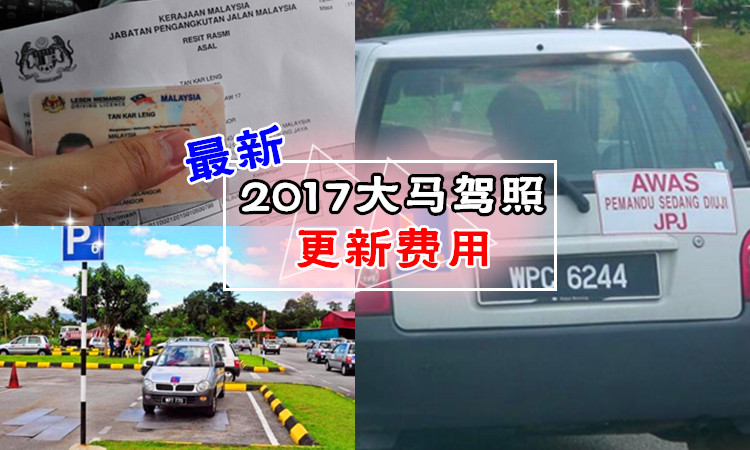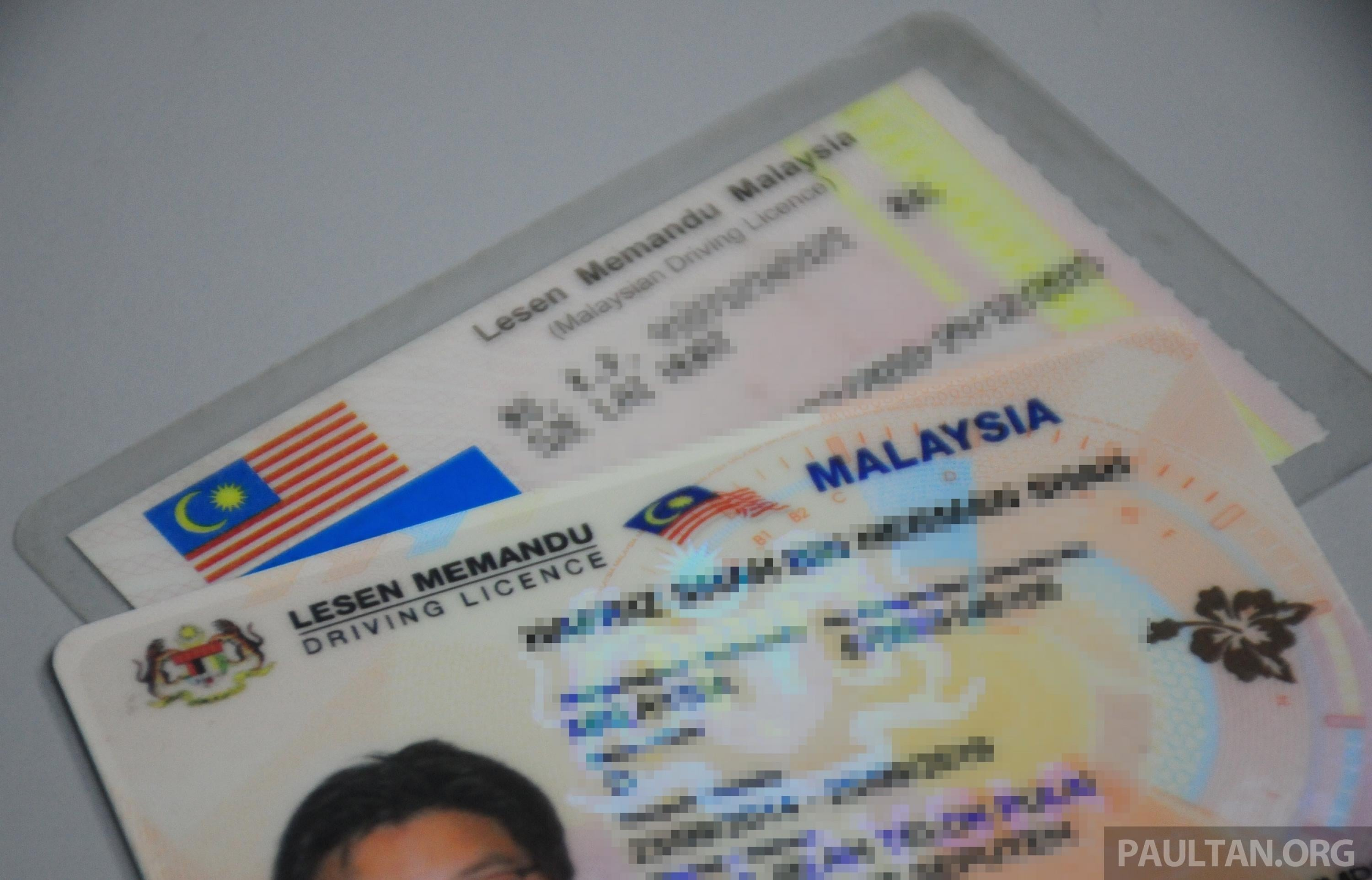A = 残疾人士摩托车（不超过450kg）

A1 = 残疾人士摩托车（超过450kg）

B = 摩托车（超过500sp）

B1 = 摩托车（不超过500sp）

B2 = 摩托车（不超过250sp）

C = 三轮车

D = 汽车（不超过3500kg）

DA =自动档汽车（不超过3500kg）

E =汽车（超过7500kg）

E1 =汽车（不超过7500kg）

E2 =汽车（不超过5000kg）

F = 重型机械（不超过5000kg）

G =重型机械（不超过5000kg）

H =重型机械（超过5000kg）

I =重型机械（超过5000kg）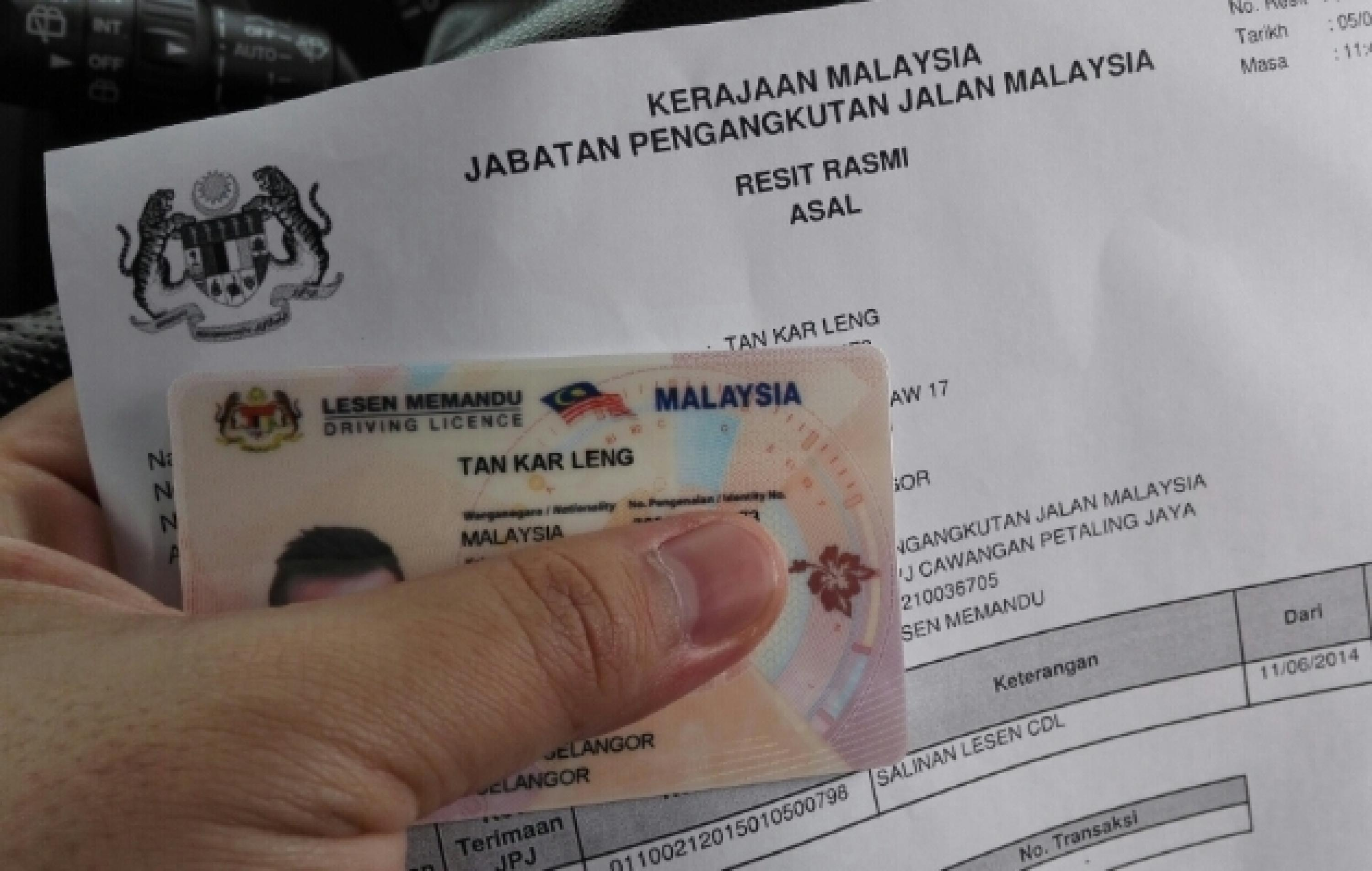LESEN BELAJAR MEMANDU (LDL) = 学车时L执照

LESEN PERCUBAAN (PDL) = 试用期的P执照

LESEN MEMANDU KOMPETEN (CDL) = 正式驾照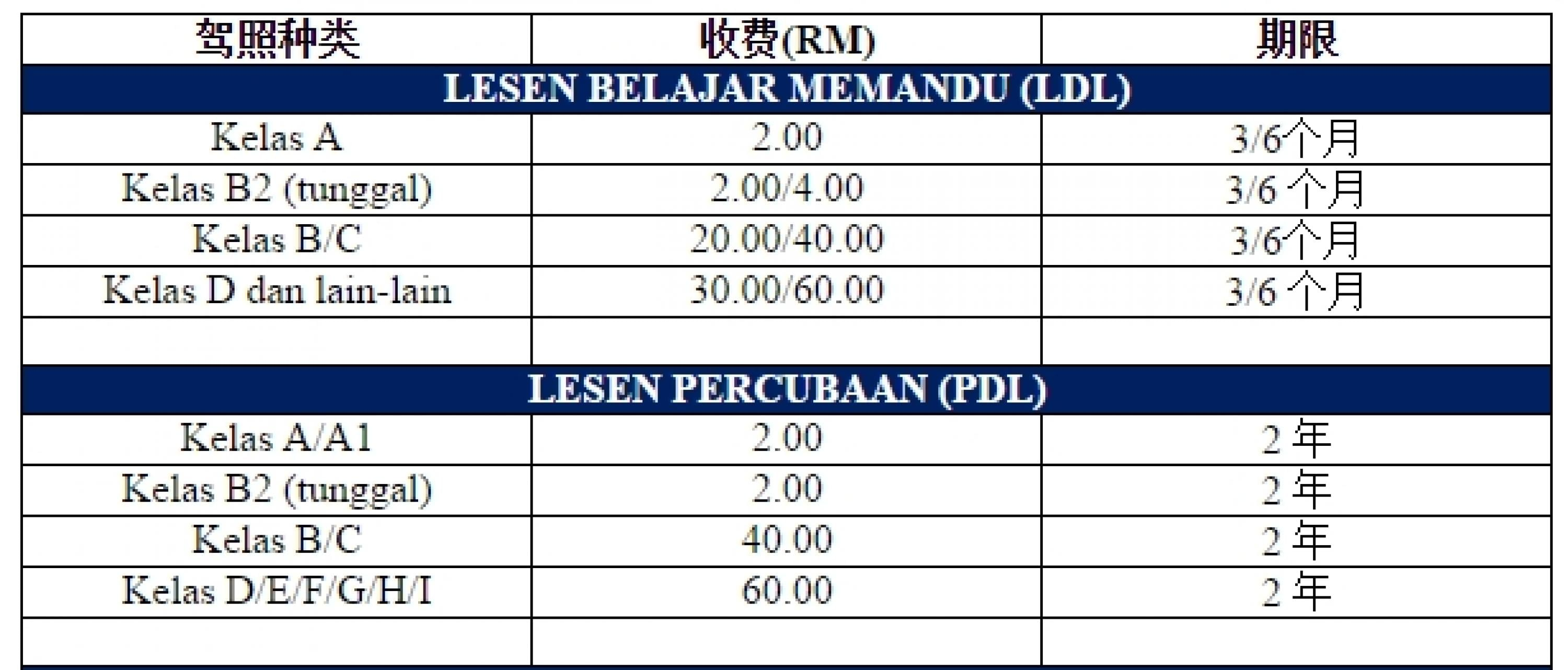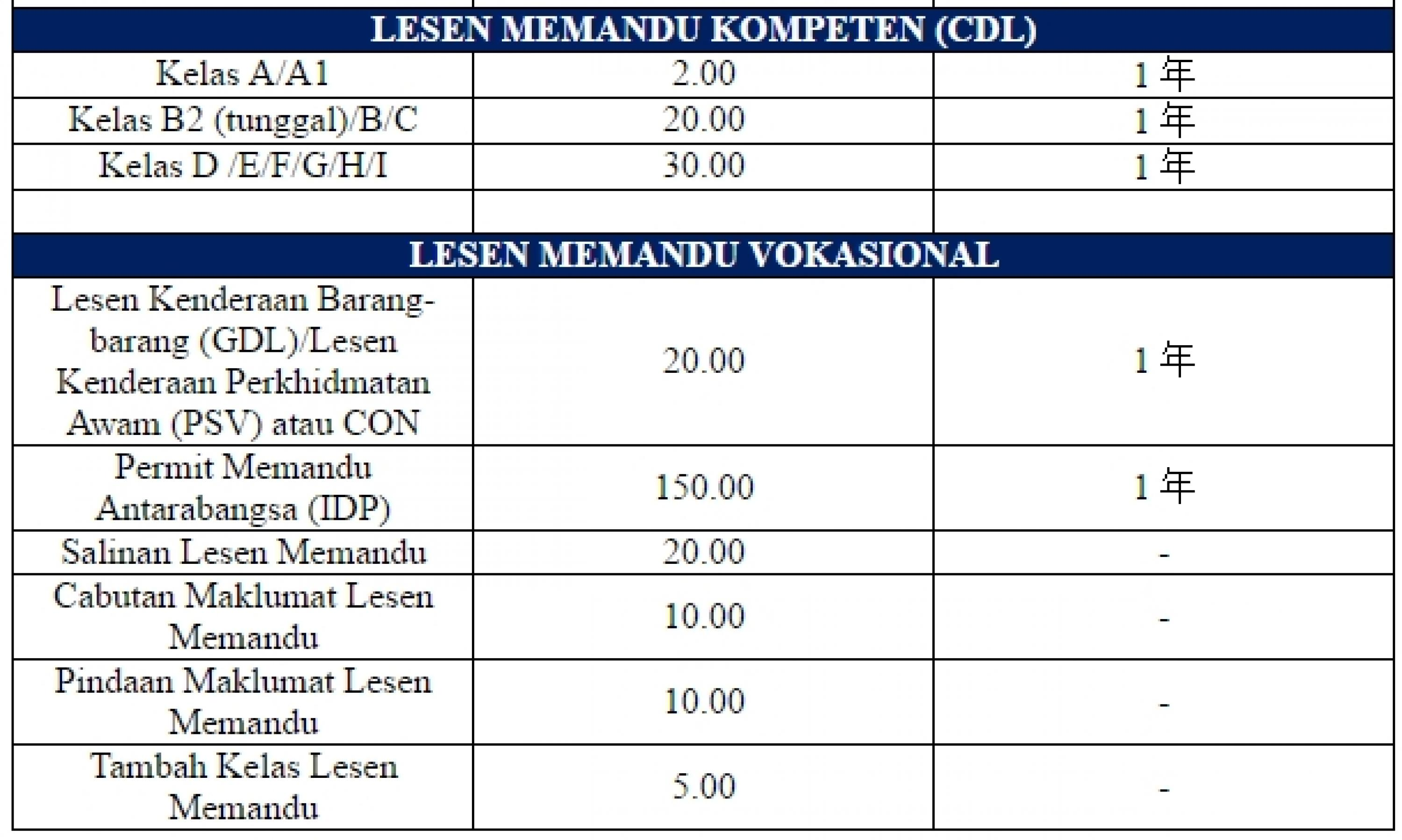Like 玩嘢兄弟团 Facebook！带大家玩翻全马high翻天～❤请点我！

Like Rojaklah Facebook！不出门也能知天下事～～请点我！

comments